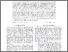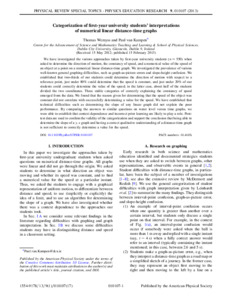# Categorization of first-year university students' interpretations of numerical linear distance-time graphs

Wemyss, T. and van Kampen, P. (2013) Categorization of first-year university students' interpretations of numerical linear distance-time graphs. Physical Review Special Topics-Physics Education Research, 9 (1).

Abstract

We have investigated the various approaches taken by first-year university students (n approximate to 550) when asked to determine the direction of motion, the constancy of speed, and a numerical value of the speed of an object at a point on a numerical linear distance-time graph. We investigated the prevalence of various well-known general graphing difficulties, such as graph-as-picture errors and slope-height confusion. We established that two-thirds of our students could determine the direction of motion with respect to a reference point, just under 80% could determine that the speed is constant, and just under 20% of our students could correctly determine the value of the speed; in the latter case, about half of the students divided the two coordinates. Three stable categories of correctly explaining the constancy of speed emerged from the data. We found that the reason given for determining that the speed of the object was constant did not correlate with successfully determining a value for the speed. We have established that technical difficulties such as determining the slope of any linear graph did not explain the poor performance. By comparing the answers to similar questions on water level versus time graphs, we were able to establish that context dependence and incorrect prior learning are likely to play a role. Post-test data are used to confirm the validity of the categorization and support the conclusion that being able to determine the slope of a y, x graph and having a correct qualitative understanding of a distance-time graph is not sufficient to correctly determine a value for the speed. DOI: 10.1103/PhysRevSTPER.9.010107

Documents
3850:1696Preview
Wemyss and van Kampen 2013.pdf